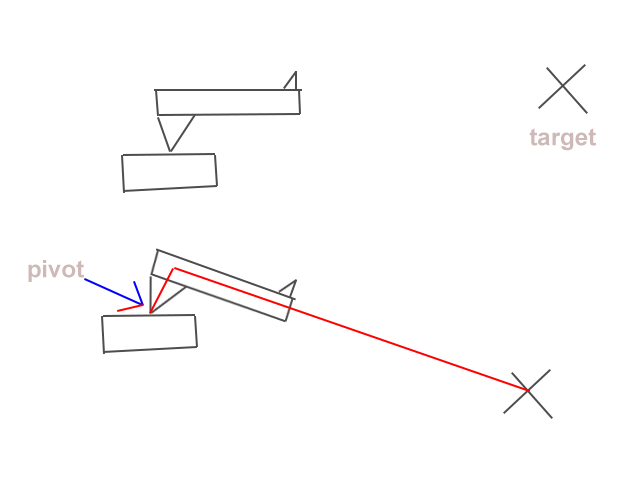# Simple LookAt Rotation with offset pivot

Hi there

This should be simple and common enough but I can’t for the life of me figure out how to do this.

I have a turret that needs to “LookAt” a target but it also has an offset pivot, so using Quaternion.LookAt( target.position ); makes the pivot look at the target resulting in the turret aiming slightly above the target.

What I need is a way of achieving the aiming illustrated in bottom drawing of my expertly drawn picture.Let me start with a quick hack. Let’s assume that your target is a transform and that the dogleg measures ‘offset’ in length. You an do:

``````transform.LookAt(target);
pos = target.position;
pos -= transform.up * offset;
transform.LookAt(pos);
``````

This hack points the pivot point at the target, then it moves the target ‘down’ by the amount of the offset then aims again. This is a simple, but not perfect solution given the geometry. It should be very close if there is any significant distance between the gun and the target.

Here is a second solution that has the potential to be more accurate. It is untested, and I’m not 100% certain I got it right. It calculate the angle difference and rotates the gun around the vector3.right. I believe it will have problems if the gun has any local ‘z’ rotation.

``````transform.LookAt(target);
var dist = (target.position - transform.position).magnitude;
var angle = Mathf(offset/dist) * Mathf.Rad2Deg;
transform.rotation = Quaternion.AngleAxis(-angle, transform.right) * transform.rotation;
``````

For what it’s worth I needed a variation of this that gave me the total angle without using LookAt. This of course would not work in 3D but it was pretty effective for my needs. It also comes with the option of moving the center of the object as well, so if you had a turret image where the pivot was far right and the muzzle was far left it could handle it. Left the debug lines in so you can see how it is lining up. Thanks to aldonaletto here Is there way to find a negative angle? - Unity Answers for the get angle logic.

Code:

``````public static float GetAngle(Vector3 A, Vector3 B)
{
//Find angle and ensure we can get a negitive angle if needed
float angle = Vector3.Angle(A, B);
Vector3 cross = Vector3.Cross(A, B);
if (cross.z < 0)
{
angle = -angle;
}

return angle;
}

/// <summary>
/// Rotates the selected transform based on its pivot point to aim at the desired point
/// </summary>
/// <param name="transform"></param>
/// <param name="point"></param>
public static void AimAt(Transform transform, Vector3 pivot, Vector3 point, Vector3 center)
{
//Find pivot and center relative to transform in world space (making sure to apply rotation to pivot offset)
Vector3 piv = transform.position + (transform.rotation * pivot);
Vector3 cent = transform.position + (transform.rotation * center);

//Find vector pointing to target
Vector3 targetAim = point - cent;

float angle = GetAngle(transform.up, targetAim);
transform.RotateAround(piv, Vector3.forward, angle);

Debug.DrawRay(piv, Vector3.up * 0.5f, Color.red);
Debug.DrawRay(piv, Vector3.right * 0.5f, Color.red);

Debug.DrawRay(cent, targetAim, Color.green);
Debug.DrawRay(point, Vector3.up, Color.yellow);
Debug.DrawRay(point, Vector3.right, Color.yellow);
}
``````